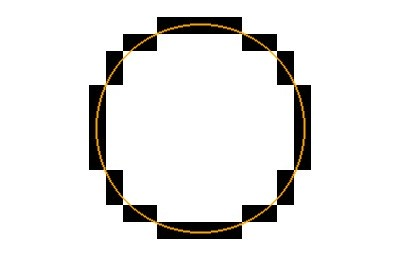Zeno's Paradox And The End Of Infinity Centuries ago, ancient Greek philosopher Zeno of Elea created a series of thought experiments collectively known as Zeno's paradoxes, each designed to prove that motion is illusory. The most intractable of these is the dichotomy paradox, an experiment that concerns infinity. This simple thought experiment leads to the conclusion that time, space and matter is quantized, or in other words that at some stage an indivisible object is present in everything that can be divided. Moreso, that this applies to any mathematics that attempts to explain the universe too. That has big implications; infinity does not exist, even in mathematics. I am unsure of the practical implications of this. The Dichotomy Paradox "That which is in locomotion must arrive at the half-way stage before it arrives at the goal."  Aristotle, Physics VI:9, 239b10 In short, if a path of motion can be divided into an infinite number of steps then an infinite amount of time would be needed to traverse it. Therefore motion cannot exist. Motion does exist however, so something must be incorrect. Let's look at a similar example applied to an object. If I take a rod, and cut it in half, then cut each half in half, and keep repeating, I can continue forever. Therefore the log is infinitely long, because no matter how small each division is, if it has some length but an infinite number of divisions then the length is infinite. We now know that this is not true because, at a small scale, objects are made of indivisible objects called atoms. For this purpose atoms as as good as indivisible here because although atoms can be smashed, the parts that they are made of eventually disintegrate into particles of zero dimension. Thus the rod is not of infinite length, because there are a finite number of atoms and each atom contains a finite and quantifiable amount of energy. In effect, the dichotomy paradox is a theoretical proof of the existence of atoms. And, when applied to distance as in the motion example, the same analogy proves the existence of something like an atom but of distance, an indivisible unit of distance. The dichotomy paradox shows that spacetime is quantized. So, the problem of infinities proves the need for quantum mechanics. Infinities in nature are eliminated by quantum mechanisms; activities on the tiniest scale that are either present or not-present, occurring or not-occurring, but never divisible. Now, this has an impact on mathematics for if infinity cannot occur in nature then in should not occur in maths. When it comes to applying maths practically, it is easily understood that infinity cannot occur; a computer could never hold an infinite number and a mind cannot imagine an infinite line, only a theoretically infinite computer or mind could do those things. The finite and infinity are incompatible. It may also be true, it appears undeniable, that any system with infinities cannot contain finites and vice versa. A mathematical description of the universe that involves infinity would describe an infinite and ideal universe, not the real universe. Ideally a new mathematics is called for, one with no infinities. What difference would this mathematics have to a mathematics with infities? One difference is that an increase in accuracy of input variables will, at some point, not influence the accuracy of the output variables. Take for example, pi, a number with an infinite number of digits. To calculate the circumference of a circle one multiplies pi with the diameter. According to mathematics, the more accurate the value of pi the more accurate the measurement would be but if the universe is quantized then this is not the case, and at some specific point adding more digits to pi will not affect the result.This can be illustrated in example above. The circle is 36 black quantized units in circumference. Increased accuracy in the ideal orange circle would not increase the accuracy of the measurement of the black circle.

 Mark Sheeky, 31 Dec 2007